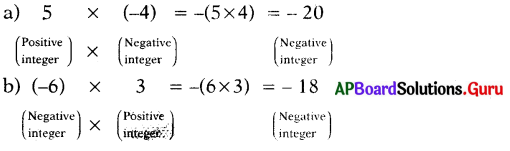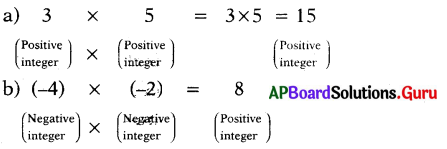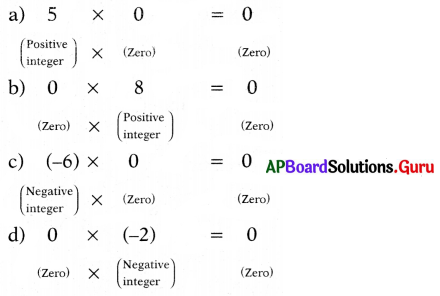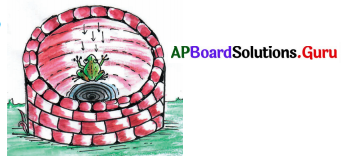# AP Board 7th Class Maths Solutions Chapter 1 Integers Ex 1.1

SCERT AP 7th Class Maths Solutions Pdf Chapter 1 Integers Ex 1.1 Textbook Exercise Questions and Answers.

## AP State Syllabus 7th Class Maths Solutions 1st Lesson Integers Exercise 1.1

Question 1.
Multiply the following.
(i) 5 × 7
5 × 7 = 35(ii) (-9) × (6) .
(-9) × (6)
= -(9 × 6) = – 54

(iii) (9) × (-4)
9 × – 4
= – (9 × 4) = – 36

(iv) (8) × (-7)
8 × (-7) = – (8 × 7) = – 56

(v) (-124) × (-1)
(-124) × (-1) = +(124 × 1) = + 124

(vi) (-12) × (-7)
(-12) × (-7) = + (12 × 7) = + 84

(vii) (-63) × (7)
(-63) × (7) = -(63 × 7) = – 441

(viii) (7) × (-15)
7 × (-15) = – (7 × 15) = – 105Question 2.
Which is greater?
(i) 2 × (-5) or 3 × (-4)
2 × (-5) or 3 × (-4)
– (2 × 5) or – (3 × 4)
– 10 or – 12
– 10 greater than – 12
∴(-10) > (-12)

(ii) (-6) × (-7) or (-8) × 5
(-6) × (-7) or (-8) × 5
6 × 7 or -(8 × 5)
42 or – 40
42 greater than – 40
∴ 42 > (-40)

(iii) (- 6) × 10 or (- 3) × (- 21)
(-6) × 10 or (- 3) × (-21)
– (6 × 10) or 3 × 21
– 60 or 63
(- 60) less than 63
(or)
63 greater than (-60)
∴ 63 > (- 60)

(iv) 9 × (-11) or 6 × (-16)
9 × (-11) or 6 × (-16)
-(9 × 11) or – (6 × 16)
(-99) or (- 96)
(- 99) less than (- 96)
(- 99) < (- 96)
(or)
(- 96) greater than (- 99)
∴ (-96) > (-99)(v) (-8) × (-5) or (-9) × (-4)
(-8) × (-5) or (-9) × (-4) + (8 × 5) or + (9 × 4)
40 or 36
40 greater than 36
∴ 40 > 36

Question 3.
Write the pair of integers whose product will give
(i) A negative integer(ii) A positive integer(iii) ZeroQuestion 4.
A frog is slipping into a well from upper surface at a rate of 3 meters per minute, after 5 minutes what is the position of the frog in the well?No. of meters slipped by frog per minute = 3m = – 3 m
No. of meters slipped by frog per 5 minutes = (-3) × 5
= – (3 × 5)
= – 15 m
That is the frog is 15 m down from the upper surface.

Question 5.
During the summer, the level of water in a pond decreases by 5 inches every week due to evaporation. What is the change in the level of the water over a period of 6 weeks ?Decrease in water level per every week = 5 inches = (- 5)
Decrease in water level per 6 weeks = (-5) × 6 .
= – (5 × 6)
= – 30 inches
Change in the level of the water in the pond per 6 weeks is 30 inches decreased.Question 6.
A shop keeper earns a profit of ₹ 5 on one note book and loss of ₹ 3 on one pen by selling in the month of July. He sells 1500 books and 1500 pens. Find out what is his profit or loss.
Profit on each notebook = ₹5
Profit on 1500 notebooks = 1500 × 5
Total profit on 1500 note books = ₹ 75 007/-
Loss on each pen = ₹3
Which we denoted by – 3.
Loss on 1500 pens = 1500 × -3
= – ₹ 4500
profit > loss
So, he will get profit.
Total profit = 7500 – 4500
= ₹ 3000/-

Question 7.
A cement company earns a profit of ₹ 8 per bag of white cement and a loss of per bag of grey cement by selling.
The company sells 2,000 bags of white cement and 3,000 bags of grey cement in a month. Find out what is its profit or loss.
Profit on each white cement bag = ₹ 8
Profit on each white cement bag
= 2000 × 8
= ₹ 16000
Loss on each grey cement bag = ₹ 6
Which we denoted by – 6.
Loss on 3000 grey cement bags = 3000 × – 6
= – ₹ 18000
Loss is more than profit.
So, he will get loss.
∴ Total loss = 16000 + (-18000)
= + 16000 – 18000
= – 2000/-Question 8.
Fill in the blanks with suitable integer to make the statement true.
(i) (-4) × _________ = – 20
(- 4) × 5 = – 20
(- 4) × 5 = – 20
– (4 × 5) = – 20
– 20 = – 20

(ii) __________ × 5 = – 35
-7 × 5 = – 35
(-7) × 5 = – 35
– (7 × 5) = – 35
– 35 = – 35

(iii) (-6) × __________ = 48
(-6) × (-8) = 48
(-6) × (-8) = 48
6 × 8 = 48
48 = 48

(iv) __________ × (- 9) = 45
(-5) × (-9) = 45
(-5) × (-9) = 45
5 × 9 = 45
45 = 45

(v) ___________ × 7 = – 42